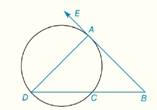Chapter 6.CR, Problem 8CR### Elementary Geometry for College St...

6th Edition
Daniel C. Alexander + 1 other
ISBN: 9781285195698

#### Solutions

Chapter
Section### Elementary Geometry for College St...

6th Edition
Daniel C. Alexander + 1 other
ISBN: 9781285195698
Textbook Problem
1 views

# In Review Exercises 5 to 10, B A → is tangent to the circle at point A in the figure shown. m ∠ D = 40 ° ,   m D C ^ = 130 ° ,     m ∠ B = ?To determine

To find: mB

Explanation

Theorem:

1) The measure of an angle formed when two secants intersect at a point outside the circle is one-half the difference of the measures of two intercepted arcs.

2) The measure of an inscribed angle of a circle is one-half the measure of its intercepted arc.

Calculation:

Given that

mD=40°, mDC^=130° and BA is tangent to the circle at point A.

By theorem, The measure of an inscribed angle ADC of a circle is one-half the measure of its intercepted arc mAC^.

40°=12mAC^

40°×2=12mAC^×2

80°=mAC^

Therefore, mAC^=80°

### Still sussing out bartleby?

Check out a sample textbook solution.

See a sample solution

#### The Solution to Your Study Problems

Bartleby provides explanations to thousands of textbook problems written by our experts, many with advanced degrees!

Get Started

#### In Exercises 31-34, evaluate h(2), where h = g f. 31. f(x) = x2 + x + 1; g(x) = x2

Applied Calculus for the Managerial, Life, and Social Sciences: A Brief Approach

#### For

Study Guide for Stewart's Multivariable Calculus, 8th

#### Distinguish between science and pseudoscience.

Research Methods for the Behavioral Sciences (MindTap Course List)